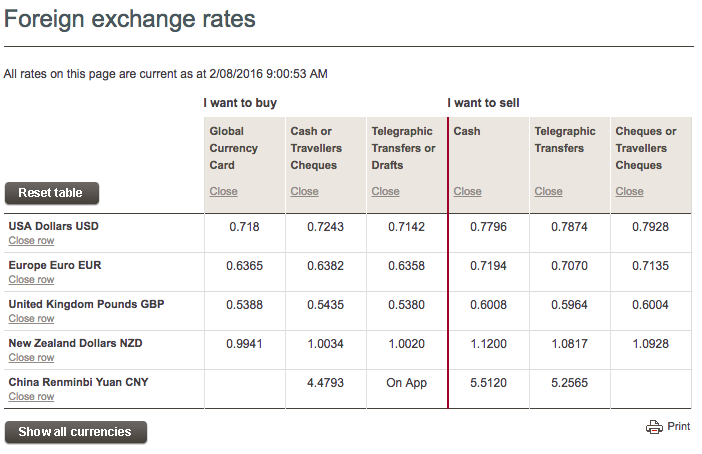# Westpac Exchange Rates Explained and Compared

This article will explain and compare the different exchange rates provided for Travel Money (cash), Travel Cards and International Money Transfers. It will help you:

1. Find the right exchange rate in your situation
2. How to use the exchange rates to calculate your currency conversion
3. Compare the exchange rates at Westpac to the rest of the options available in Australia

## Westpac currency exchange rates explainedWhen you look at the above table of exchange rates, it's easy to get confused. This is because exchange rate you receive will depend on a few things:

2. Whether you buying or selling
3. Your product (Travel card, cash or bank transfer)

Find the currency you want to buy or sell. If you can't find it, click "Show all currencies". Keep in mind that the bank does not buy and sell every currency in the world. If you are not sure about what currency you'll need for the country you are going to, check out our handy travel money guides here.

### Buying and Selling

Next, you need to work out which column of exchange rates relates to your situation.

If you are sending money overseas or travelling overseas and need to buy currency, make sure you look at the 3 columns on the left hand side marked "I want to buy". If you are receiving money from overseas or if you need to sell currency, make sure you look at the 3 columns marked "I want to sell".

Once you've worked out the right set of columns, you need to work out which product relates to you:

• "Telegraphic Transfers or Drafts" This is the exchange rate that you'll recieve if you are making a bank transfer to or from another bank account overseas. A Draft is an international bank draft which is another word for a bank cheque.
• "Global Currency Card" is the exchange rate you'll receive if you are loading a Global Currency Card
• "Cash" is the exchange rate you'll receive if you are buying or selling currency in cash via the branch

## Calculating Currency Conversions

Once you've found the right exchange rate, next comes calculating the currency conversion. To help you, here are some common situations and how you calculate the conversion:

### Buying Travel Money: How much Currency will my money buy?

If you have a set amount of money you want to convert, here is how you calculate how much currency you will receive:

The Australian Dollars You Have X (multiplied by) The Exchange Rate = Currency You Will Receive

Let's say you have \$1,000 and you are buying US dollars at a rate of 0.7180 then the amount of US dollars you will receive is

1 000 multiplied by 0.7243 = 724.30 US dollars

### How much will it cost to buy a certain amount of currency?

If you have a set amount of currency you want to buy, here is how you calculate how much it will cost in Australian dollars:

The Australian Dollar Cost = The Amount of Currency You Want to Buy / (divided by) The Exchange Rate

If you want to buy 2 000 Euros and the exchange rate is 0.6382 then to total Australian dollar cost will be

2 000 divided by 0.6382 = \$3,133.81

### How much will it cost to send a certain amount of currency overseas?

If you have a set amount of currency you want to send money overseas, the calculation is the same as buying travel money

The Australian Dollar Cost = The Amount of Currency You Want to Send / (divided by) The Exchange Rate

If you want to send 10 000 Euros and the exchange rate is 0.6358 then to total Australian dollar cost will be

10 000 divided by 0.6358 = \$15,728.22

### What about receiving currency from overseas?

If you are receiving currency from overseas, this is how to calculate how much you should receive in Australian dollars

The Australian Dollar You Receive = The Amount of Currency Received / (divided by) The Exchange Rate

If you received 10 000 British Pounds through a bank transfer and the exchange rate was 0.5964, then the amount you'd receive is:

10 000 divided by 0.5964 = \$16,767.27

## Westpac Exchange Rates Compared

The Currency Shop compares exchange rates on travel money and international money transfers: